• pythonround函数
万次阅读 多人点赞
2019-01-24 13:00:59

round(number,num_digits)

Number 需要进行四舍五入的数字。
Num_digits 指定的位数，按此位数进行四舍五入。

注解
如果 num_digits 大于 0，则四舍五入到指定的小数位。
如果 num_digits 等于 0，则四舍五入到最接近的整数。
如果 num_digits 小于 0，则在小数点左侧进行四舍五入。

示例

x=1.343671234
print x
print round(x,1)
print round(x,2)
print round(x,3)


输出结果为：

1.343671234
1.3
1.34
1.344

更多相关内容
• ## Pythonround函数

千次阅读 2020-12-28 22:29:13
round( number ) 函数会返回浮点数 number 的四舍五入值。具体定义为 round(number[,digits])：如果 digits>0 ，四舍五入到指定的小数位；如果 digits=0 ，四舍五入到最接近的整数；如果 digits<0 ，则在...

round( number ) 函数会返回浮点数 number 的四舍五入值。

具体定义为 round(number[,digits])：如果 digits>0 ，四舍五入到指定的小数位；

如果 digits=0 ，四舍五入到最接近的整数；

如果 digits<0 ，则在小数点左侧进行四舍五入；

如果 round() 函数只有 number 这个参数，则等同于 digits=0。

示例如下：

logging.info(round(9.315,2))

logging.info(round(9.3151,2))

logging.info(round(9.316,2))

logging.info(round(9.316,-1))

运行结果：

INFO - 9.31

INFO - 9.32

INFO - 9.32

INFO - 10.0注意： round(9.315,2)=9.31，并不是我们想的那样！只有 9.315 后面还有数字，才会进位，比如 round(9.3151,2)=9.32。

以上是 python3.x 的 round 函数说明。注意： python2.x 的 round 函数与 python 3.x 的 round 函数结果不同！

python2.x round 函数的官方定义为：Values are rounded to the closest multiple of 10 to the power minus ndigits; if two multiples are equally close, rounding is done away from 0. 即如果舍入处理的值，离左右两端相同距离，那么会远离 0，即为 1，所以 round(0.5)=1.0，而 round(-0.5)=-1。

python3.x round 函数的官方定义为：“values are rounded to the closest multiple of 10 to the power minus ndigits; if two multiples are equally close, rounding is done toward the even choice. 即如果舍入处理的值，离左右两端相同距离，那么会朝向偶数方向处理，所以 round(0.5)=1.0，而 round(-0.5)=1，也是 1！

展开全文• 最近在刷NCT的PYTHON等级考试题目，在使用round这个非常不起眼的四舍五入常规函数时候发觉了一件很有意思的事情，即在某些时候.5并不会五入，反而变成了四舍。具体看下如下两个例子 在这两个例子我发觉很奇怪的...

首先来简单介绍下round这个函数。

1. 语法

round( x [, n]  )

2. 参数

• x -- 数值表达式。
• n -- 数值表达式，表示从小数点位数。

3. 返回值

返回浮点数x的四舍五入值。

4. 实例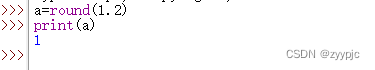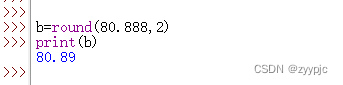最近在刷NCT的PYTHON等级考试题目，在使用round这个非常不起眼的四舍五入常规函数时候发觉了一件很有意思的事情，即在某些时候.5并不会五入，反而变成了四舍。具体看下如下两个例子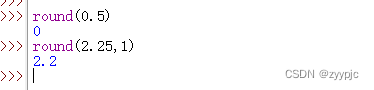在这两个例子我发觉很奇怪的现象，照道理0.5四舍五入应该是1，而2.25保留一位的四舍五入应该是2.3，而实际得到的结果与我们的数学尝试都不相符，这是为什么呢？？？

查阅了Python的文档后，发现这个问题其实是由Python版本所导致。这个问题只存在于Pyhon3中，而Python2中则一切正常。我们先来看下如下文档的描述。

在python2.7的doc中，round()的最后写着，"Values are rounded to the closest multiple of 10 to the power minus ndigits; if two multiples are equally close, rounding is done away from 0." 保留值将保留到离上一位更近的一端（四舍六入），如果距离两端一样远，则保留到离0远的一边。所以round(0.5)会近似到1，而round(-0.5)会近似到-1。

但是到了python3.5的doc中，文档变成了"values are rounded to the closest multiple of 10 to the power minus ndigits; if two multiples are equally close, rounding is done toward the even choice." 如果距离两边一样远，会保留到偶数的一边。比如round(0.5)和round(-0.5)都会保留到0，而round(1.5)会保留到2。

紧接着我们分别在Python2/3中做实验去验证一下

版本:Python 3.10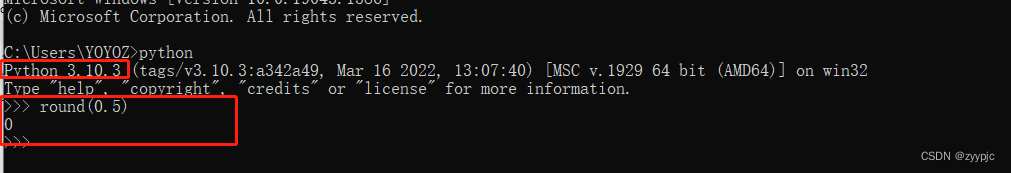版本:Python 2.7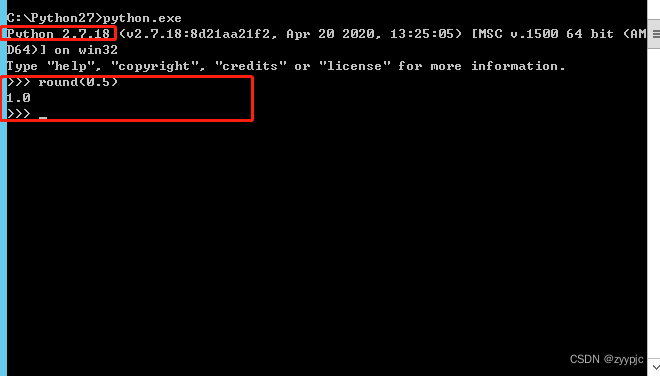# 所以如果有项目是从python2.x迁移到python3.x的，可要注意一下round的地方（当然，还要注意/和//，还有print，还有一些比较另类的库）！！！！！！

### 基于目前存在的这种情况，尽量避免使用round函数，我通过网络整理了下如下可供大家参考

• 使用math模块中的一些函数，比如math.ceiling（天花板除法）。
• python自带整除，python2中是/，3中是//，还有div函数。
• 字符串格式化可以做截断使用，例如 "%.2f" % value（保留两位小数并变成字符串……如果还想用浮点数请披上float()的外衣）。
• 当然，对浮点数精度要求如果很高的话，请用嘚瑟馍，不对不对，请用decimal模块。
展开全文python
• 分享了Python中的精确小数计算。decimal模块及round函数的四舍五入经常遇见的问题。

# 前言🥦

在Python官方有这样一句话:The behavior of round() for floats can be surprising: for example, round(2.675, 2) gives 2.67 instead of the expected 2.68. This is not a bug: it’s a result of the fact that most decimal fractions can’t be represented exactly as a float. See Floating Point Arithmetic: Issues and Limitations for more information也就是说,在Python语言中小数的存储往往是近似值,并不像整数那样精确。我们呢有时候需求就是对小数的指定位数进行四舍五入,今天博主在这里分享一下在Python中进行小数四舍五入的几种方法。如果你也有什么好的方法欢迎评论区留言哦。

# 一、小数不精确的常见问题🥦

Python中的小数存储往往是不精确的,相加的时候可能会导致精度丢失，或者结果出乎意料。

## 1.相加🌴

print(1.01+1.02)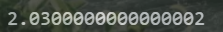## 2.四舍五入🌴

以下代码的打印结果
print(round(1.135,2))
print(round(1.235,2))
print(round(1.335,2))
print(round(1.435,2))
print(round(1.535,2))
print(round(1.635,2))
最后的一位数应该都为4，但是由于精度丢失,导致有些数四舍五入失败。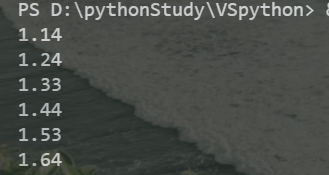# 二、round函数🥦

## 1.简介🌴

官方给出的接口如下：

def round(number: SupportsRound[_T], ndigits: SupportsIndex)


第一个参数指的是需要进行四舍五入的小数,第二个参数是保留的小数位
默认是保留0位小数,也就是保留到整数。

## 2.使用方法🌴

### ① 代码🌵：

print(round(1.135,2))
print(round(1.235,2))
print(round(1.335,2))
print(round(1.435,2))
print(round(1.535,2))
print(round(1.635,2))


### ② 运行结果🌵：### ③ 暴露问题🌵：

可以看出来运行结果不是很理想,因为有些数值四舍五入进位失败，我们可以处理一下,然后就可以得到准确的结果了。经过分析当只有一位小数的时候保留到整数,进位比较准确。
代码：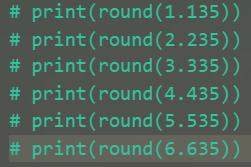结果：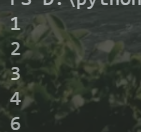### ④ 解决方法🌵：

我们可以对代码做出这样的改动,也就是利用四舍五入一位小数可以获得精确值的性质
可以先将数值扩大n倍,直到将保留小数位的下一位小数提到小数部分第一位,也就是说保留两位小数就将第三位小数提到第一位,做的操作就是乘以100。依次类推

print(round(1.235,2))
print(round(1.3355*100)/100)
print(round(1.435,2))
print(round(1.5355*100)/100)
print(round(1.635,2))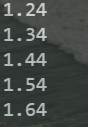# 三、Decimal模块🥦

这是一个精确小数计算的模块,咱们只在这里说一下他的简单使用方法。

## 1.创建Decimal小数🌴

### ① 字符串型🌵

代码：

print("Decimal精确的1.35：",Decimal("1.35"))


运行结果：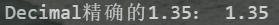### ② 数值型🌵

代码：

print("float型的1.35",Decimal(1.35))


运行结果：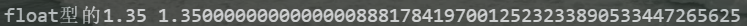## 2.结合round函数精确四舍五入🌴

代码：

from decimal import Decimal
print(round(Decimal("1.535"),2))
print(round(Decimal("1.335"),2))


运行结果：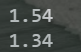## 3.使用自身方法精确四舍五入🌴

代码：

print(Decimal("1.3355").quantize(Decimal("0.000")))
print(Decimal("1.2955").quantize(Decimal("0.00")))
print(Decimal("1.3355").quantize(Decimal("0.00")))


运行结果：展开全文python decimal
• Python round()函数描述round() 方法返回浮点数x的四舍五入值。语法以下是 round() 方法的语法:参数x -- 数值表达式。返回值返回浮python
• 解决Python round函数，奇进偶不进的问题 def newRound(data,num): import math try: data = float(data) except : return "非数字不能处理" return int((data * math.pow(10,int(num)) + 0.5))/math.pow(10,...python
• 关于round函数的详细用法和一些由于机器数问题产生的错误情况 round(number[, ndigits]) 返回number舍入到小数点后ndigits为精度的值，如果ndigits被省略则会返回最接近输入值的整数python
•python
• round函数是一个用于四舍五入的函数；在python3中，round(1.0/2.0)得到的是1，而在python2中，round(1.0/2.0)得到的是0。例如\$ pythonPython 2.7.8 (default, Jun 18 2015, 18:54:19)[GCC 4.9.1] on linux2Type ...
• ## Python中round函数

千次阅读 2021-11-02 11:51:59
Pythonround函数Python round()描述语法参数实例补充round函数并不总是四舍五入参数n Python round() 描述 返回浮点数 x 的四舍五入值 语法 round(x[, n]) 参数 x：数值表达式 n：数值表达式，表示精确到...python
• 在EXCEL中ROUND这个函数怎么用语法ROUND(number,num_digits)Number 需要进行四舍五入的数字。Num_digits 指定的位数，按此位数进行四舍五入。说明如果 num_digits 大于 0，则四舍五入到指定的小数位。如果 num_...
• ## pythonround函数用法

万次阅读 2016-11-25 13:48:27
round()方法返回 x 的小数点四舍五入到n个数字。语法 以下是round()方法的语法：round( x [, n] ) 参数 x –这是一个数值表达式n –这也是一个数值表达式返回值 该方法返回 x 的小数点四舍五入到n个数字例子 ...python
• 使用round函数的时候发现了一个奇怪的现象。...Python版本问题Python2和 Python 3有很多实现细节上的不同，round函数是其中不同之一。在Python3中，round(1.0/2.0)得到的是1，而在Python2中，round(1.0/2...
• round函数很简单，对浮点数进行近似取值，保留几位小数。比如 >>> round(10.0/3, 2) 3.33 >>> round(20/7) 3 第一个参数是一个浮点数，第二个参数是保留的小数位数，可选，如果不写的话默认保留到整数。 这么简单的...
• ## 详解python中的round()函数

万次阅读 多人点赞 2022-03-02 18:27:11
round()是python自带的一个函数，用于数字的四舍五入。 使用方法（环境python3） round(number,digits) 参数： digits>0，四舍五入到指定的小数位 digits=0, 四舍五入到最接近的整数 digits<0 ，在小数点左侧...python
• ## python中 round函数的使用

万次阅读 多人点赞 2020-12-15 17:22:55
round(参数，保留的小数位)，该函数遵循四舍六入五成偶原则 例如1： print(round(3.545,2)) 输出结果： 3.54 例如2: print(round(3.5455,2)) 输出结果： 3.55
• round( number ) 函数会返回浮点数 number 的四舍五入值。 具体定义为 round(number[,digits])： 如果 digits>0 ，四舍五入到指定的小数位； 如果 digits=0 ，四舍五入到最接近的整数； 如果 digits<0 ，则在...
• python中使用的语法是round...如果round函数只有参数number,等同于digits 等于 0 返回的值是四舍五入后的值 其四舍五入的规矩一般为如下： （1）要求保留位数的后一位如果是4或者4以下的数字，则舍去， 例如 5.214保留
• round函数概念： 英文：圆，四舍五入是python内置函数，它在哪都能用，对数字取四舍五入。 round(number[, ndigits]) round 对传入的数据进行四舍五入，如果ngigits不传，默认是0（就是说保留整数部分）.ngigits&...
• ## round函数

千次阅读 2020-12-05 06:00:24
Python内置的round函数，用来取小数点后N位，还是很方便的。但是要注意，round函数执行的不是四舍五入。请参考：Python实现四舍五入的方法。看代码吧，要注意round函数在去小数点后N位时，N可以是负数：>>>...
• Python 3.6.1 (default, Sep 7 2017, 16:36:03) [GCC 6.3.0 20170406] on linux Type "help", "copyright", "credits" or "license" for more information. >>> round(3.5) 4 >>> round(3.55, .....
• 最近在Python中使用round()函数取整然后后处理乘以100时，发现了一个奇怪的问题，如下： >>> round(61 / 154 * 100, 2) 39.61 >>> >>> (round(61 / 154, 2) )* 100 40.0 >>> ...python round函数
• python中使用round函数的方法发布时间：2020-08-06 11:50:31
• 函数的列表参数属于形参 矩阵.shape=行，列
• round函数很简单，对浮点数进行近似取值，保留几位小数。比如>>> round(10.0/3, 2)3.33>>> round(20/7)3第一个参数是一个浮点数，第二个参数是保留的小数位数，可选，如果不写的话默认保留到整数...
• round函数很简单(而且不需要引入math模块)，对浮点数进行近似取值，保留几位小数。比如#-*- coding: UTF-8 -*-r1=round(12.12345,3)r2=round(12.12345)print r1,' ',r2结果1、round的结果跟python版本有关我们来看看...
• python内置函数——round()获取浮点数的四舍五入值python
• round()函数：此函数慎用！！！ 作用：返回浮点数x的“奇进偶舍值”。 round() 方法的语法: round( x [, n] ) 参数： x – 数值表达式。 n – 数值表达式，表示保留小数点后的位数。 返回值： 返回浮点数x的“奇进偶...
• 我用的最多的就是round(a,b)函数，其中a是输入的数值，b就是要保留的为数，你想保留2位，b就是2，想保留3位，b就是3 example： a = 3.234534 b = round(a,2) print(b) ## 输出的就是3.23 #或者是下面这样一种...python...